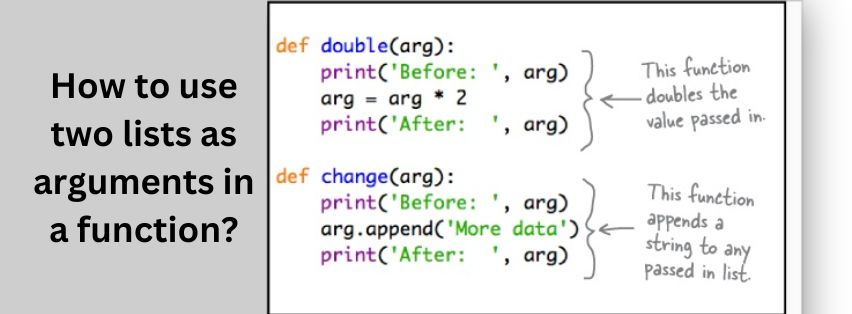Jully 18, 2022

### How to use two lists as arguments in a function?

Using two lists as arguments in a function can be a powerful tool for programming. It allows for the efficient and effective manipulation of data and can be used to solve complex problems. This essay will discuss how to use two lists as arguments in a function, including how to define the function, how to pass the lists as arguments, and how to use the lists within the function.

### Defining the Function

When using two lists as arguments in a function, the first step is to define the function. This includes specifying the parameters, which are the variables that will be used within the function. For example, if the function is to add two lists together, the parameters would be two lists, such as list1 and list2. The parameters should be defined in the function definition, such as “def add_lists(list1, list2):”.

### Passing the Lists as Arguments

Once the function is defined, the next step is to pass the lists as arguments. This can be done by calling the function with the two lists. For example, if the function is called “add_lists” and the two lists are “list1” and “list2”, then the call would be “add_lists(list1, list2)”. This will pass the two lists to the function.

### Using the Lists Within the Function

Once the two lists have been passed to the function, they can then be used within the function. This can be done by accessing the elements of each list and performing operations on them. For example, if the function is to add two lists together, then each element of each list can be accessed and added together. This can be done using a loop such as a for loop, which will iterate through each element of each list and add them together.

### Returning a Result

Once all of the elements of each list have been added together, a result can be returned from the function. This can be done by using a return statement at the end of the function. For example, if the result of adding two lists together is a new list, then a return statement such as “return new_list” can be used to return the new list from the function.

### Error Handling

When using two lists as arguments in a function, it is important to consider error handling. This includes checking for errors such as invalid inputs or empty lists. If an error is detected, then appropriate action should be taken such as returning an error message or raising an exception. This will ensure that any errors are handled properly and that the program does not crash.

### Conclusion:

Using two lists as arguments in a function can be a powerful tool for programming. It allows for efficient and effective manipulation of data, and can be used to solve complex problems. The process of using two lists as arguments in a function includes defining the function, passing the lists as arguments, using the lists within the function, returning a result, and handling errors. By following these steps, it is possible to use two lists as arguments in a function and achieve powerful results.

### Recent Posts### Discuss the different types of booking systems and their benefits.

Jully 11, 2022